# RD Sharma Solutions for Class 6 Maths Chapter 2: Playing with Numbers Exercise 2.7

## RD Sharma Solutions for Class 6 Maths Exercise 2.7 PDF

Some of the students are unable to understand concepts during class hours and hence struggle to score marks. They feel shy to get their doubts cleared in class, and as a consequence, have less knowledge about the concepts. In order to help them overcome this issue and improve self studying methods, experts at BYJU’S provide exercise wise solutions. This mainly boosts confidence among students to perform well in the exam. The PDF of solutions can be used as a reference material to improve efficiency in solving problems. RD Sharma Solutions for Class 6 Maths Chapter 2 Playing with Numbers Exercise 2.7 are provided here.

## RD Sharma Solutions for Class 6 Maths Chapter 2: Playing with Numbers Exercise 2.7 Download PDF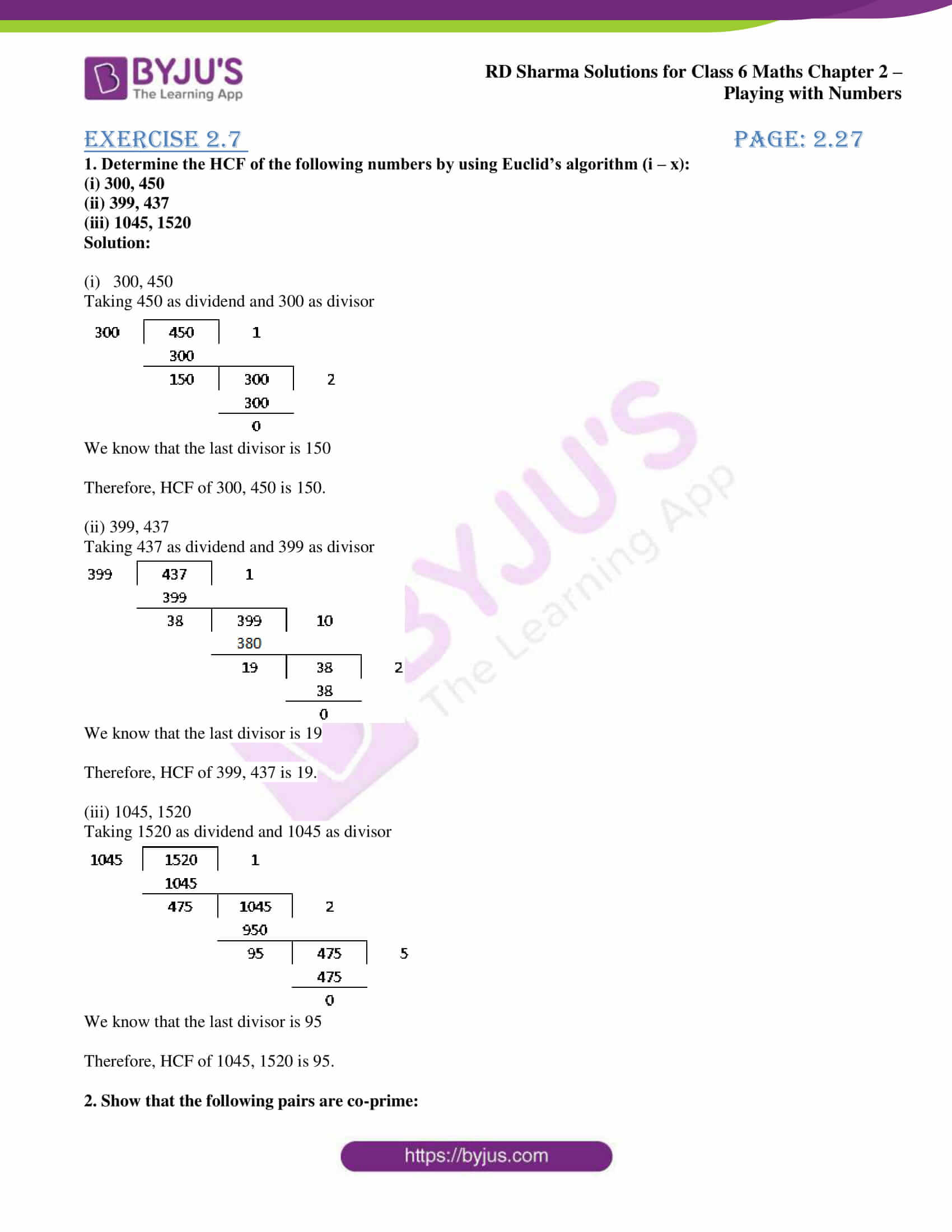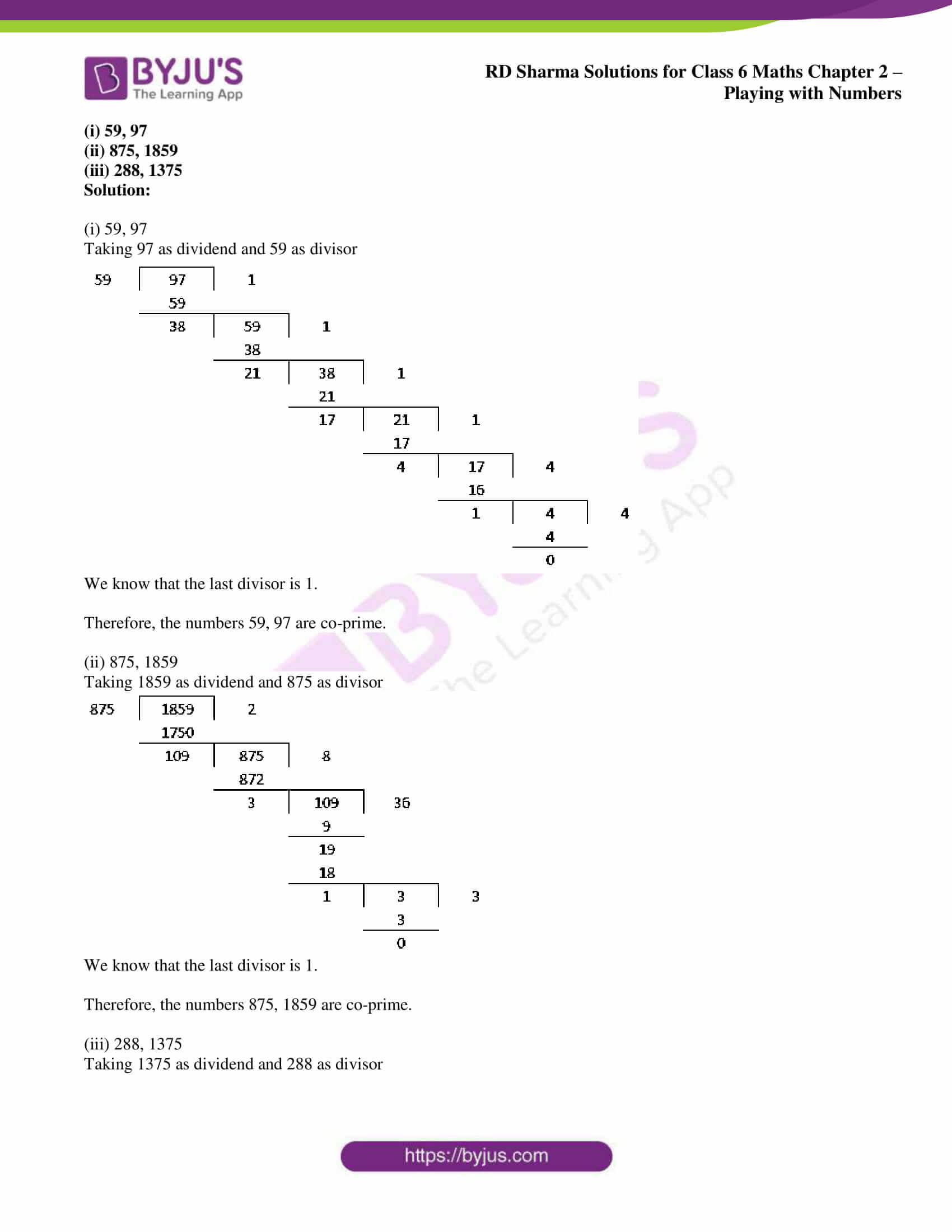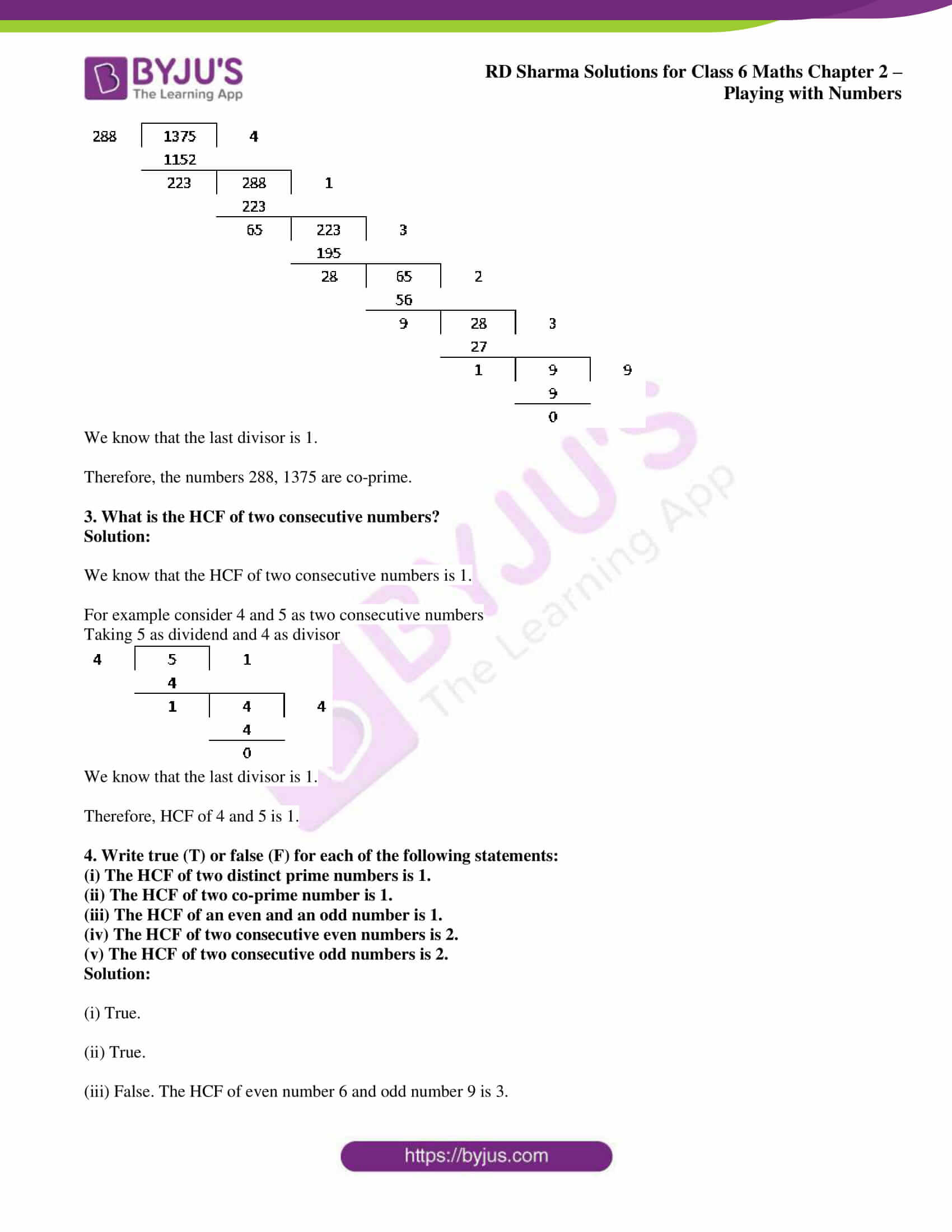## Access answers to Maths RD Sharma Solutions for Class 6 Chapter 2: Playing with Numbers Exercise 2.7

1. Determine the HCF of the following numbers by using Euclid’s algorithm (i – x):

(i) 300, 450

(ii) 399, 437

(iii) 1045, 1520

Solution:

(i) 300, 450

Taking 450 as dividend and 300 as divisor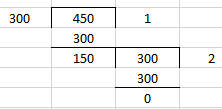We know that the last divisor is 150

Therefore, HCF of 300, 450 is 150.

(ii) 399, 437

Taking 437 as dividend and 399 as divisor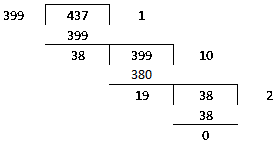We know that the last divisor is 19

Therefore, HCF of 399, 437 is 19.

(iii) 1045, 1520

Taking 1520 as dividend and 1045 as divisor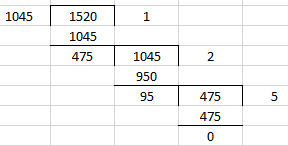We know that the last divisor is 95

Therefore, HCF of 1045, 1520 is 95.

2. Show that the following pairs are co-prime:

(i) 59, 97

(ii) 875, 1859

(iii) 288, 1375

Solution:

(i) 59, 97

Taking 97 as dividend and 59 as divisor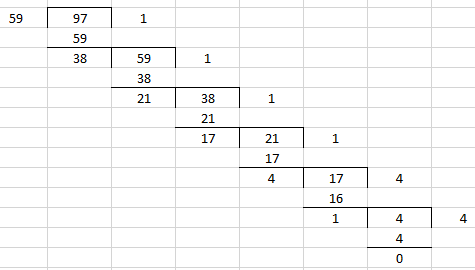We know that the last divisor is 1.

Therefore, the numbers 59, 97 are co-prime.

(ii) 875, 1859

Taking 1859 as dividend and 875 as divisor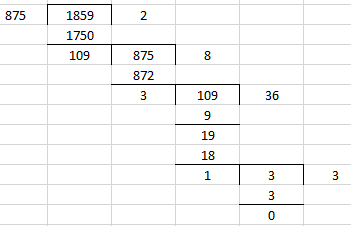We know that the last divisor is 1.

Therefore, the numbers 875, 1859 are co-prime.

(iii) 288, 1375

Taking 1375 as dividend and 288 as divisor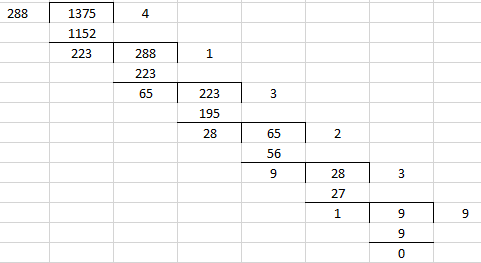We know that the last divisor is 1.

Therefore, the numbers 288, 1375 are co-prime.

3. What is the HCF of two consecutive numbers?

Solution:

We know that the HCF of two consecutive numbers is 1.

For example consider 4 and 5 as two consecutive numbers

Taking 5 as dividend and 4 as divisor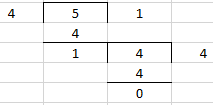We know that the last divisor is 1.

Therefore, HCF of 4 and 5 is 1.

4. Write true (T) or false (F) for each of the following statements:

(i) The HCF of two distinct prime numbers is 1.

(ii) The HCF of two co-prime number is 1.

(iii) The HCF of an even and an odd number is 1.

(iv) The HCF of two consecutive even numbers is 2.

(v) The HCF of two consecutive odd numbers is 2.

Solution:

(i) True.

(ii) True.

(iii) False. The HCF of even number 6 and odd number 9 is 3.

(iv) True.

(v) False. The HCF of numbers 25 and 27 is 1.

### RD Sharma Solutions for Class 6 Maths Chapter 2 – Playing with Numbers Exercise 2.7

RD Sharma Solutions Class 6 Maths Chapter 2 Playing with Numbers Exercise 2.7 contains concepts like Euclid’s Algorithm and methods of finding HCF for larger numbers.

### Key features of RD Sharma Solutions for Class 6 Maths Chapter 2: Playing with Numbers Exercise 2.7

• RD Sharma Solutions are created based on the CBSE syllabus and guidelines for Class 6.
• The solutions are provided for all the exercises which are covered in each chapter to help students perform well in the exam.
• It helps students to obtain a clear idea about the concepts and self evaluate conceptual knowledge of each chapter.
• The primary aim of providing solutions is to bridge the gap between students and education.